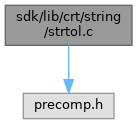ReactOS  0.4.15-dev-4916-gd519b11
strtol.c File Reference
`#include <precomp.h>`
Include dependency graph for strtol.c:Go to the source code of this file.

## Functions

long CDECL strtol (const char *nptr, char **endptr, int base)

## ◆ strtol()

 long CDECL strtol ( const char * nptr, char ** endptr, int base )

Definition at line 8 of file strtol.c.

9 {
10  const char *s = nptr;
11  unsigned long acc;
12  int c;
13  unsigned long cutoff;
14  int neg = 0, any, cutlim;
15
16  /*
17  * Skip white space and pick up leading +/- sign if any.
18  * If base is 0, allow 0x for hex and 0 for octal, else
19  * assume decimal; if base is already 16, allow 0x.
20  */
21  do {
22  c = *s++;
23  } while (isspace(c));
24  if (c == '-')
25  {
26  neg = 1;
27  c = *s++;
28  }
29  else if (c == '+')
30  c = *s++;
31  if ((base == 0 || base == 16) &&
32  c == '0' && (*s == 'x' || *s == 'X'))
33  {
34  c = s;
35  s += 2;
36  base = 16;
37  }
38  if (base == 0)
39  base = c == '0' ? 8 : 10;
40
41  /*
42  * Compute the cutoff value between legal numbers and illegal
43  * numbers. That is the largest legal value, divided by the
44  * base. An input number that is greater than this value, if
45  * followed by a legal input character, is too big. One that
46  * is equal to this value may be valid or not; the limit
47  * between valid and invalid numbers is then based on the last
48  * digit. For instance, if the range for longs is
49  * [-2147483648..2147483647] and the input base is 10,
50  * cutoff will be set to 214748364 and cutlim to either
51  * 7 (neg==0) or 8 (neg==1), meaning that if we have accumulated
52  * a value > 214748364, or equal but the next digit is > 7 (or 8),
53  * the number is too big, and we will return a range error.
54  *
55  * Set any if any `digits' consumed; make it negative to indicate
56  * overflow.
57  */
58  cutoff = neg ? ((unsigned long)LONG_MAX+1) : LONG_MAX;
59  cutlim = cutoff % (unsigned long)base;
60  cutoff /= (unsigned long)base;
61  for (acc = 0, any = 0;; c = *s++)
62  {
63  if (isdigit(c))
64  c -= '0';
65  else if (isalpha(c))
66  c -= isupper(c) ? 'A' - 10 : 'a' - 10;
67  else
68  break;
69  if (c >= base)
70  break;
71  if (any < 0 || acc > cutoff || (acc == cutoff && c > cutlim))
72  any = -1;
73  else
74  {
75  any = 1;
76  acc *= base;
77  acc += c;
78  }
79  }
80  if (any < 0)
81  {
82  acc = neg ? LONG_MIN : LONG_MAX;
83 #ifndef _LIBCNT_
85 #endif
86  }
87  else if (neg)
88  acc = 0-acc;
89  if (endptr != 0)
90  *endptr = any ? (char *)((size_t)(s - 1)) : (char *)((size_t)nptr);
91  return acc;
92 }
errno_t __cdecl _set_errno(_In_ int _Value)
#define isspace(c)
Definition: acclib.h:69
#define isalpha(c)
Definition: acclib.h:74
GLuint base
Definition: 3dtext.c:35
unsigned char
Definition: typeof.h:29
#define isdigit(c)
Definition: acclib.h:68
__kernel_size_t size_t
Definition: linux.h:237
#define isupper(c)
Definition: acclib.h:71
const GLubyte * c
Definition: glext.h:8905
#define LONG_MAX
Definition: limits.h:43
#define ERANGE
Definition: acclib.h:92
GLdouble s
Definition: gl.h:2039
#define long
Definition: qsort.c:33
#define c
Definition: ke_i.h:80
#define LONG_MIN
Definition: limits.h:42